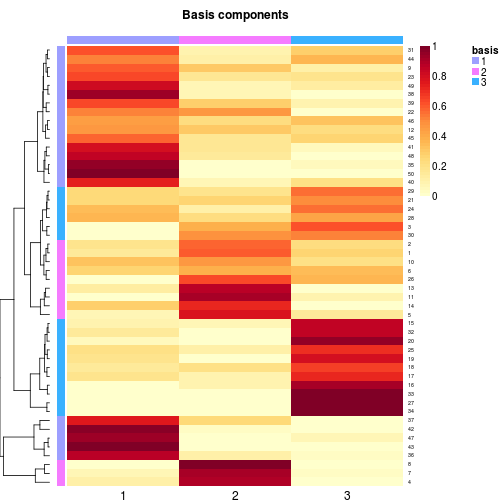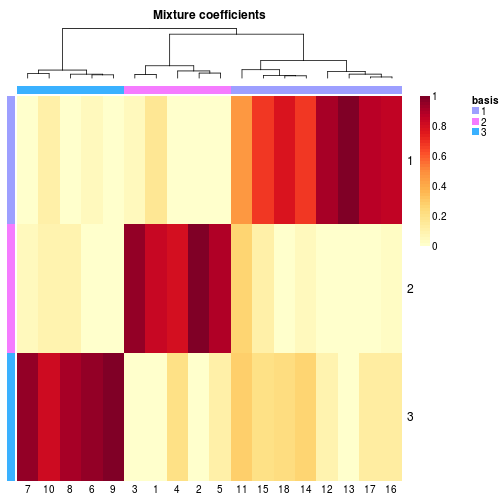# Algorithms and framework for Nonnegative Matrix Factorization (NMF).

## Description

This package provides a framework to perform Non-negative Matrix Factorization (NMF). It implements a set of already published algorithms and seeding methods, and provides a framework to test, develop and plug new/custom algorithms. Most of the built-in algorithms have been optimized in C++, and the main interface function provides an easy way of performing parallel computations on multicore machines.

## Details

`nmf` Run a given NMF algorithm

## Examples

``````
# generate a synthetic dataset with known classes
n <- 50; counts <- c(5, 5, 8);
V <- syntheticNMF(n, counts)

# perform a 3-rank NMF using the default algorithm
res <- nmf(V, 3)

basismap(res)
````````````coefmap(res)
````````````
``````

`nmf`